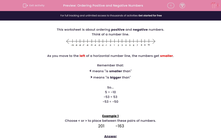# Ordering Positive and Negative Numbers

In this worksheet, students use the < and > symbols to order positive and negative numbers.This content is premium and exclusive to EdPlace subscribers.Key stage:  KS 2

Curriculum topic:   Maths and Numerical Reasoning

Curriculum subtopic:   Place Value

Difficulty level:#### Worksheet Overview

This worksheet is about ordering positive and negative numbers.

Think of a number line.As you move to the left of a horizontal number line, the numbers get smaller.

Remember that:

< means "is smaller than"

> means "is bigger than"

So...

5 > -10

-53 < 53

-53 < -50

Example 1

Choose < or > to place between these pairs of numbers.

201 -163

201 is positive.

-163 is negative.

Positive numbers are always bigger than negative numbers.

So 201 is bigger than -163.

201
 >
-163

Example 2

Choose < or > to place between these pairs of numbers.

-201 -163

-201 is negative.

-163 is negative.

Which is further to the left on the number line?

-201 is more negative, so it is smaller than -163.

So -201 is smaller than -163.

-201
 <
-163

### What is EdPlace?

We're your National Curriculum aligned online education content provider helping each child succeed in English, maths and science from year 1 to GCSE. With an EdPlace account you’ll be able to track and measure progress, helping each child achieve their best. We build confidence and attainment by personalising each child’s learning at a level that suits them.

Get started